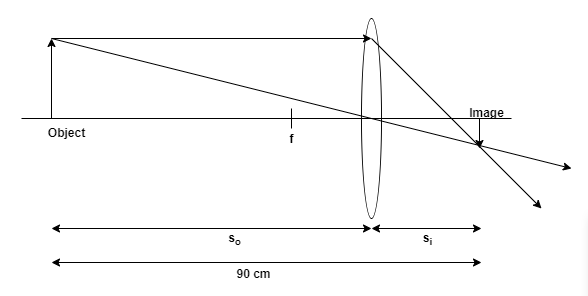# Problem: A student is working in an optics lab and has a light bulb which is 90 cm from a screen. She needs to make a real image of the bulb on the screen and she has a positive lens with a focal length of 12.5 cm. Find two places she can put the lens and give object and image distances to both setups.

###### FREE Expert Solution

Len's equation,
$\overline{)\frac{\mathbf{1}}{\mathbf{f}}{\mathbf{=}}\frac{\mathbf{1}}{{\mathbf{s}}_{\mathbf{i}}}{\mathbf{+}}\frac{\mathbf{1}}{{\mathbf{s}}_{\mathbf{o}}}}$

$\overline{){\mathbf{x}}{\mathbf{=}}\frac{\mathbf{-}\mathbf{b}\mathbf{±}\sqrt{{\mathbf{b}}^{\mathbf{2}}\mathbf{+}\mathbf{4}\mathbf{a}\mathbf{c}}}{\mathbf{2}\mathbf{a}\mathbf{b}}}$f = 12.5 cm

so = so

si = (90 - so) cm###### Problem Details

A student is working in an optics lab and has a light bulb which is 90 cm from a screen. She needs to make a real image of the bulb on the screen and she has a positive lens with a focal length of 12.5 cm. Find two places she can put the lens and give object and image distances to both setups.